Anúncio

# Step-down transformer Physics project Class 12 CBSE Final

23 de Feb de 2016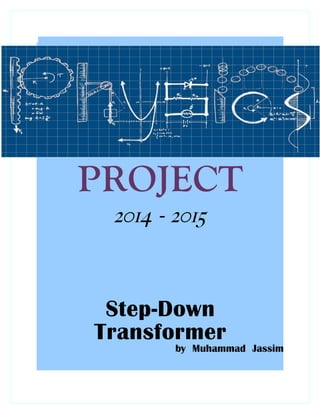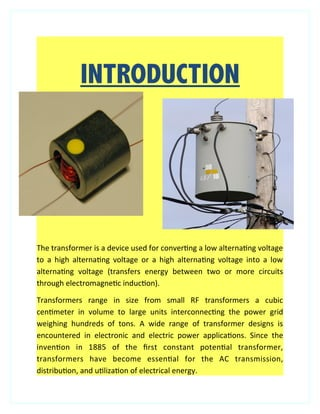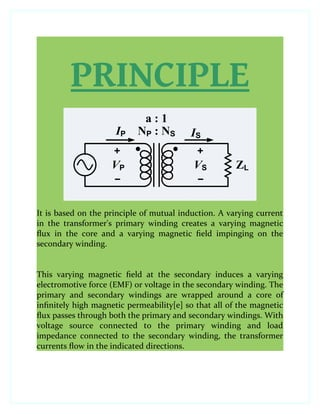Anúncio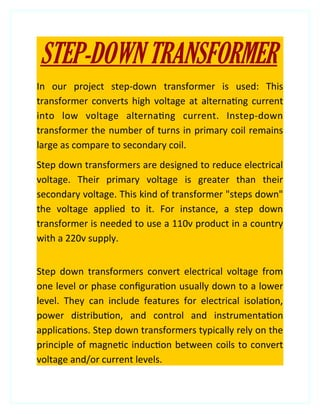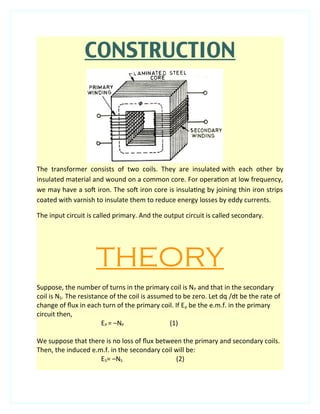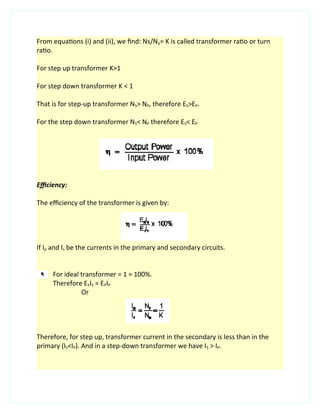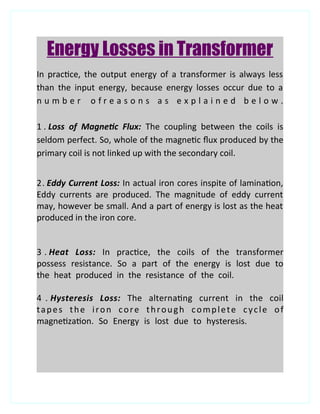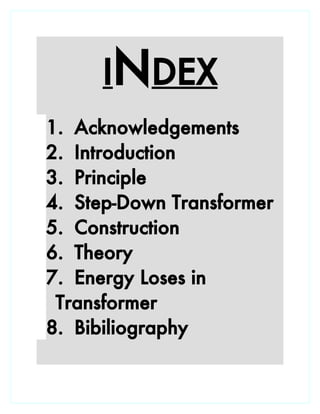AnúncioPróximos SlideShares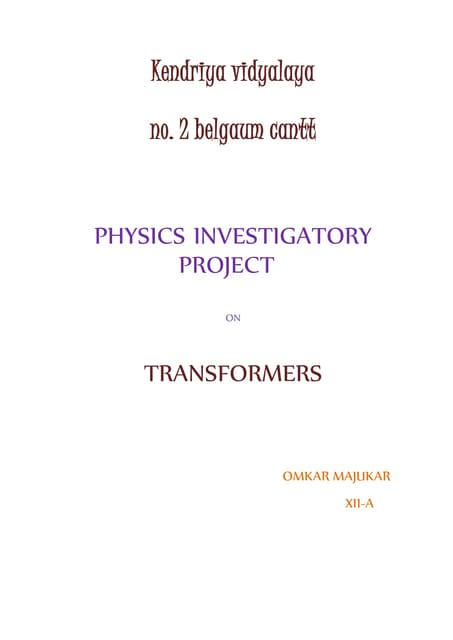Transformers
Carregando em ... 3
1 de 10
Anúncio

### Step-down transformer Physics project Class 12 CBSE Final

1. PROJECT 2014 - 2015 Step-Down Transformer by Muhammad Jassim
2. Acknowledgements "There are times when silence speaks so much more loudly than words of praise to only as good as belittle a person, whose words do not express, but only put a veneer over true feelings, which are of gratitude at this point of time." I would like to express my sincere gratitude to my physics mentor Mr. Nizar, for his vital support, guidance and encouragement, without which this project would not have come forth. I would also like to express my gratitude to the staff of the Department of Physics at Birla Public School for their support during the making of this project.
3. INTRODUCTION The transformer is a device used for convertng a low alternatng voltage to a high alternatng voltage or a high alternatng voltage into a low alternatng voltage (transfers energy between two or more circuits through electromagnetc inducton). Transformers range in size from small RF transformers a cubic centmeter in volume to large units interconnectng the power grid weighing hundreds of tons. A wide range of transformer designs is encountered in electronic and electric power applicatons. Since the inventon in 1885 of the frst constant potental transformer, transformers have become essental for the AC transmission, distributon, and utlizaton of electrical energy.
4. PRINCIPLE It is based on the principle of mutual induction. A varying current in the transformer's primary winding creates a varying magnetic fux in the core and a varying magnetic feld impinging on the secondary winding. This varying magnetic feld at the secondary induces a varying electromotive force (EMF) or voltage in the secondary winding. The primary and secondary windings are wrapped around a core of infnitely high magnetic permeability[e] so that all of the magnetic fux passes through both the primary and secondary windings. With voltage source connected to the primary winding and load impedance connected to the secondary winding, the transformer currents fow in the indicated directions.
5. STEP-DOWN TRANSFORMER In our project step-down transformer is used: This transformer converts high voltage at alternatng current into low voltage alternatng current. Instep-down transformer the number of turns in primary coil remains large as compare to secondary coil. Step down transformers are designed to reduce electrical voltage. Their primary voltage is greater than their secondary voltage. This kind of transformer "steps down" the voltage applied to it. For instance, a step down transformer is needed to use a 110v product in a country with a 220v supply. Step down transformers convert electrical voltage from one level or phase confguraton usually down to a lower level. They can include features for electrical isolaton, power distributon, and control and instrumentaton applicatons. Step down transformers typically rely on the principle of magnetc inducton between coils to convert voltage and/or current levels.
6. CONSTRUCTION The transformer consists of two coils. They are insulated with each other by insulated material and wound on a common core. For operaton at low frequency, we may have a sof iron. The sof iron core is insulatng by joining thin iron strips coated with varnish to insulate them to reduce energy losses by eddy currents. The input circuit is called primary. And the output circuit is called secondary. THEORY Suppose, the number of turns in the primary coil is NP and that in the secondary coil is NS. The resistance of the coil is assumed to be zero. Let dq /dt be the rate of change of fux in each turn of the primary coil. If Ep be the e.m.f. in the primary circuit then, EP = –NP (1) We suppose that there is no loss of fux between the primary and secondary coils. Then, the induced e.m.f. in the secondary coil will be: ES= –NS (2)
7. From equatons (i) and (ii), we fnd: Ns/Np= K is called transformer rato or turn rato. For step up transformer K>1 For step down transformer K < 1 That is for step-up transformer NS> NP, therefore ES>EP. For the step down transformer NS< NP therefore ES< EP Efciency: The efciency of the transformer is given by: If Ip and Is be the currents in the primary and secondary circuits. For ideal transformer = 1 = 100%. Therefore ESIS = EPIP Or Therefore, for step up, transformer current in the secondary is less than in the primary (IS<IP). And in a step-down transformer we have IS > IP.
8. Energy Losses in Transformer In practce, the output energy of a transformer is always less than the input energy, because energy losses occur due to a n u m b e r o f r e a s o n s a s e x p l a i n e d b e l o w . 1 . Loss of Magnetc Flux: The coupling between the coils is seldom perfect. So, whole of the magnetc fux produced by the primary coil is not linked up with the secondary coil. 2. Eddy Current Loss: In actual iron cores inspite of laminaton, Eddy currents are produced. The magnitude of eddy current may, however be small. And a part of energy is lost as the heat produced in the iron core. 3 . Heat Loss: In practce, the coils of the transformer possess resistance. So a part of the energy is lost due to the heat produced in the resistance of the coil. 4 . Hysteresis Loss: The alternatng current in the coil tapes the iron core through complete cycle of magnetzaton. So Energy is lost due to hysteresis.
9. INDEX 1. Acknowledgements 2. Introduction 3. Principle 4. Step-Down Transformer 5. Construction 6. Theory 7. Energy Loses in Transformer 8. Bibiliography
10. Bibliography BIBLIOGRAP - Internet- www.wikipedia.com, www.encylopedia.com – NCERT Physics Textbook
Anúncio